# Trigonometric Functions Of Any Angle Unit Circle Rationale

• Slides: 28Trigonometric Functions Of Any AngleUnit Circle Rationale •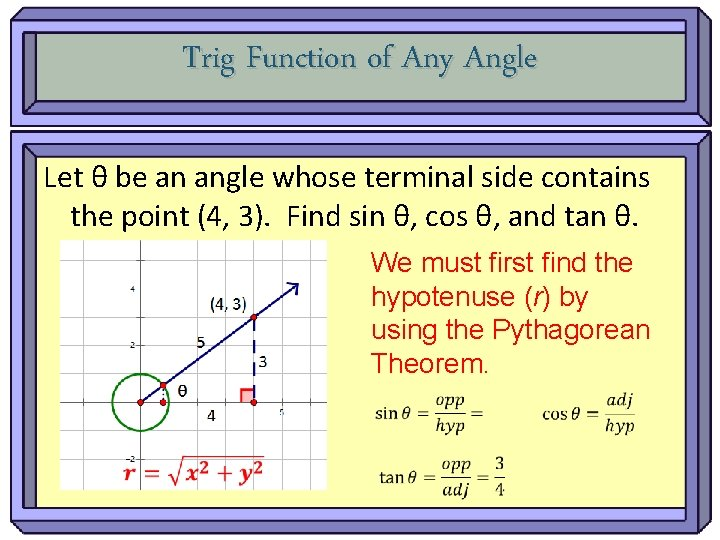Trig Function of Any Angle Let θ be an angle whose terminal side contains the point (4, 3). Find sin θ, cos θ, and tan θ. We must first find the hypotenuse (r) by using the Pythagorean Theorem.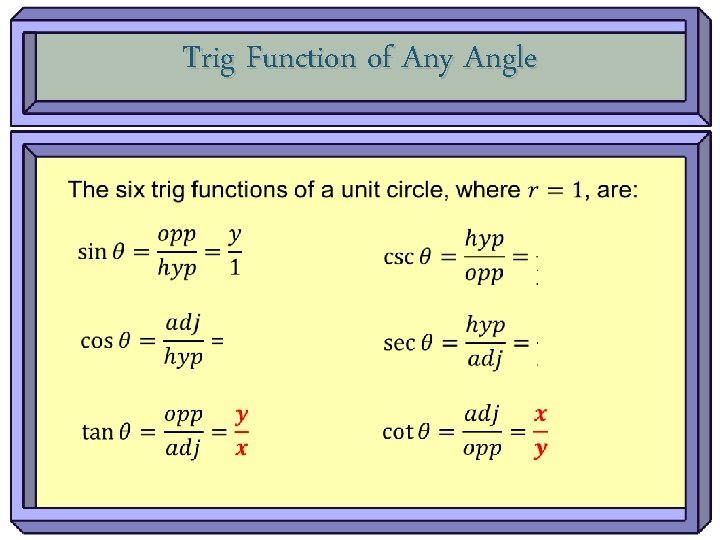Trig Function of Any AngleTrig Function of Any Angle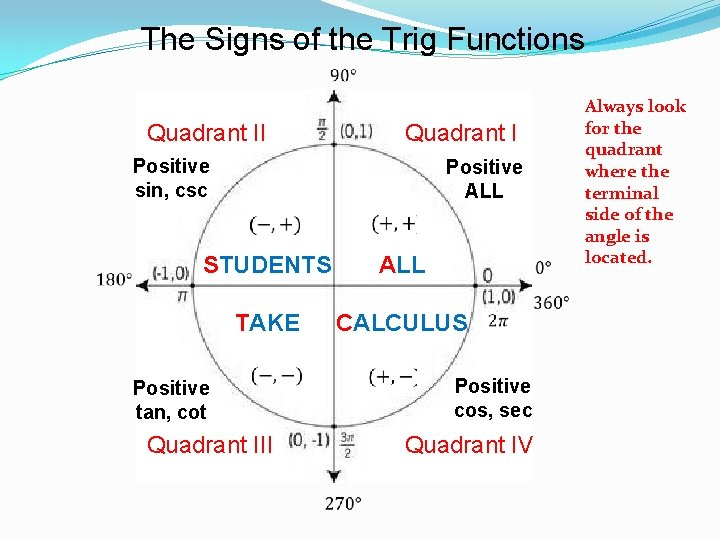The Signs of the Trig Functions Quadrant II Positive sin, csc Positive ALL STUDENTS TAKE Positive tan, cot CALCULUS Quadrant III Positive cos, sec Quadrant IV Always look for the quadrant where the terminal side of the angle is located.P Check for the validity of the signs in the answers.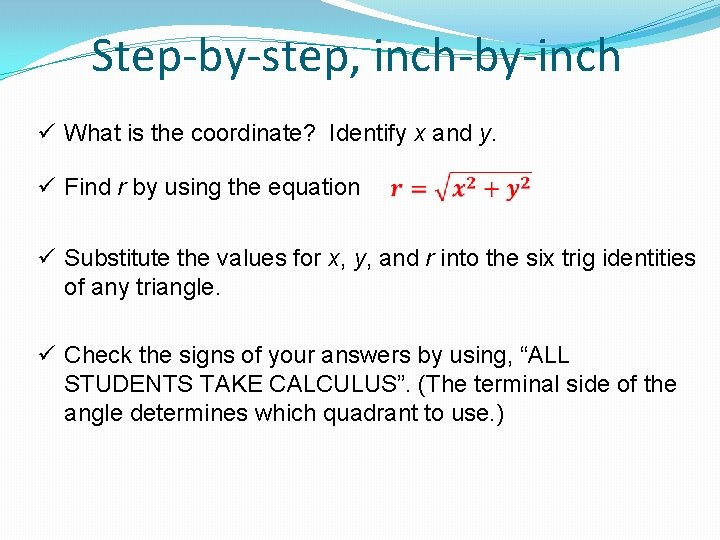Step-by-step, inch-by-inch ü What is the coordinate? Identify x and y. ü Find r by using the equation ü Substitute the values for x, y, and r into the six trig identities of any triangle. ü Check the signs of your answers by using, “ALL STUDENTS TAKE CALCULUS”. (The terminal side of the angle determines which quadrant to use. )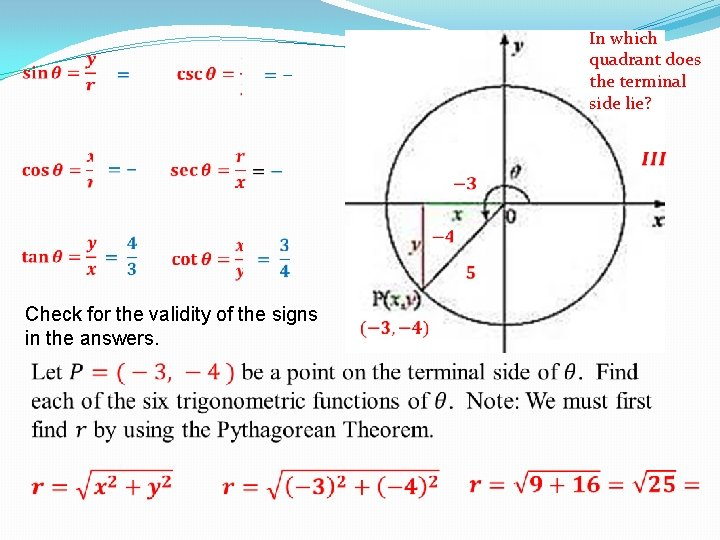In which quadrant does the terminal side lie? Check for the validity of the signs in the answers.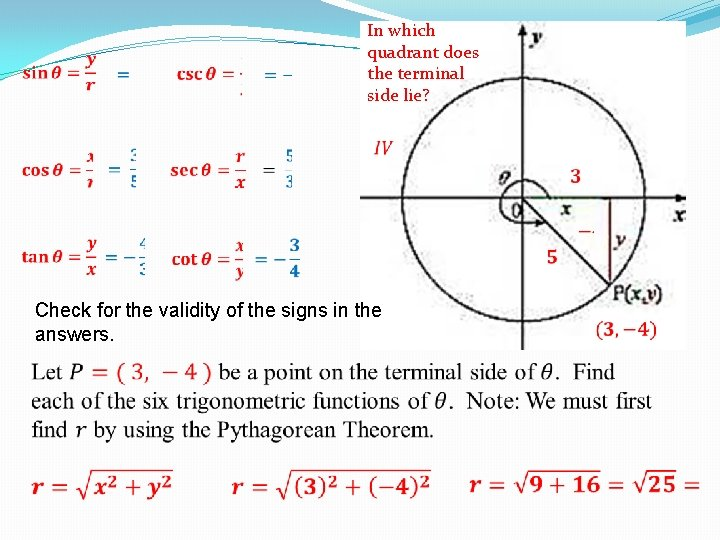In which quadrant does the terminal side lie? Check for the validity of the signs in the answers. In which quadrant does the terminal side lie?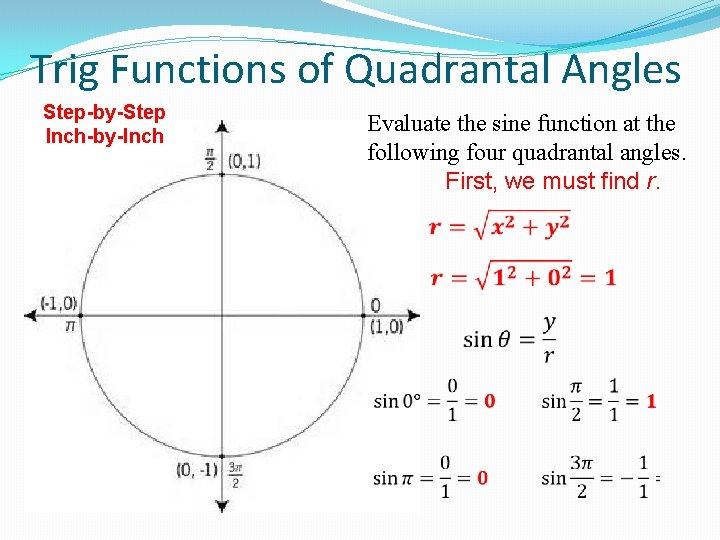Trig Functions of Quadrantal Angles Step-by-Step Inch-by-Inch Evaluate the sine function at the following four quadrantal angles. First, we must find r.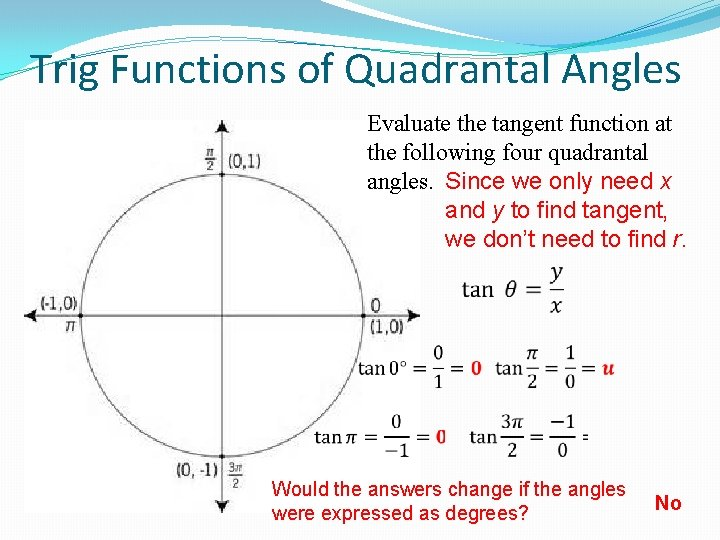Trig Functions of Quadrantal Angles Evaluate the tangent function at the following four quadrantal angles. Since we only need x and y to find tangent, we don’t need to find r. Would the answers change if the angles were expressed as degrees? No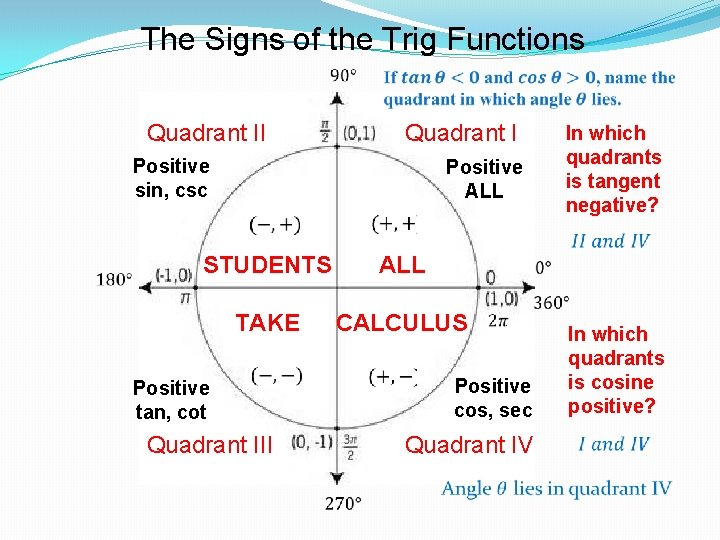The Signs of the Trig Functions Quadrant II Positive sin, csc Positive ALL TAKE ALL STUDENTS Positive tan, cot In which quadrants is tangent negative? CALCULUS Quadrant III Positive cos, sec Quadrant IV In which quadrants is cosine positive?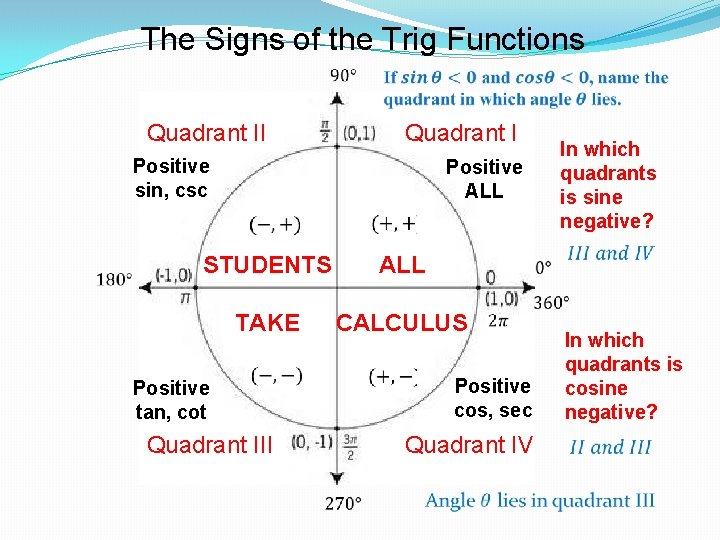The Signs of the Trig Functions Quadrant II Positive sin, csc Positive ALL STUDENTS TAKE Positive tan, cot In which quadrants is sine negative? CALCULUS Quadrant III In which quadrants is cosine negative? Positive cos, sec Quadrant IV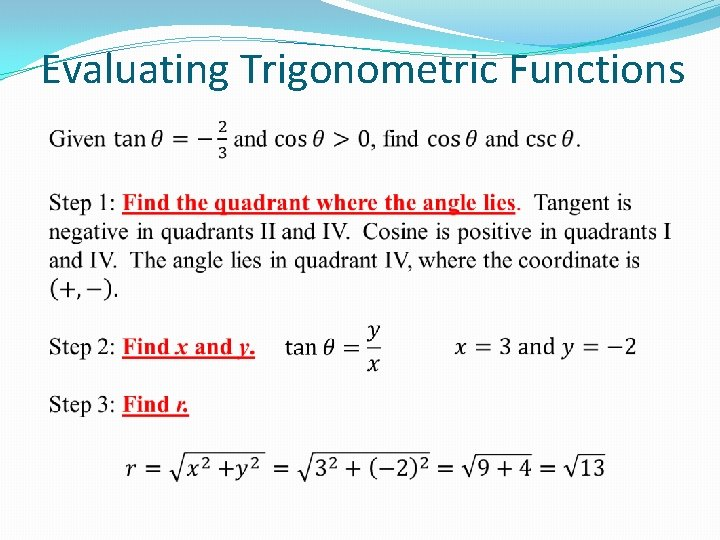Evaluating Trigonometric FunctionsEvaluating Trigonometric Functions Check: cosine is positive in quadrant IV. Check: Cosecant is negative in quadrant IV.Evaluating Trigonometric Functions Step 2: Find x and y. Step 3: Find r.Evaluating Trigonometric Functions Check: Sine is positive in quadrant II. Check: Cosecant is negative in quadrant II.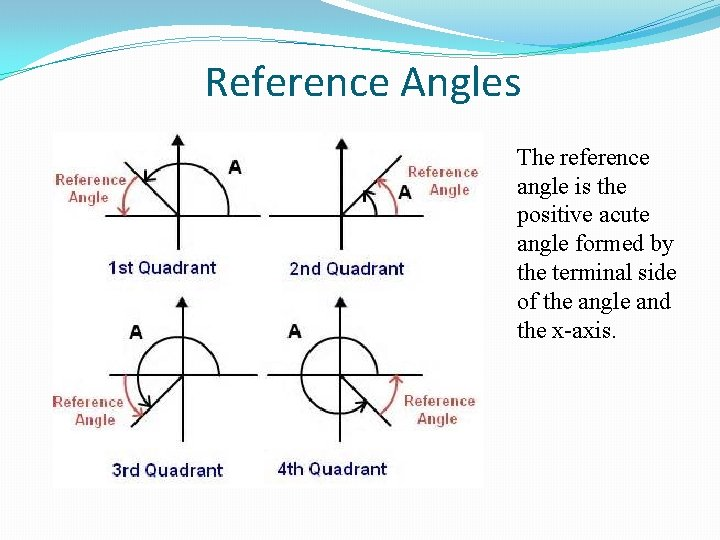Reference Angles The reference angle is the positive acute angle formed by the terminal side of the angle and the x-axis.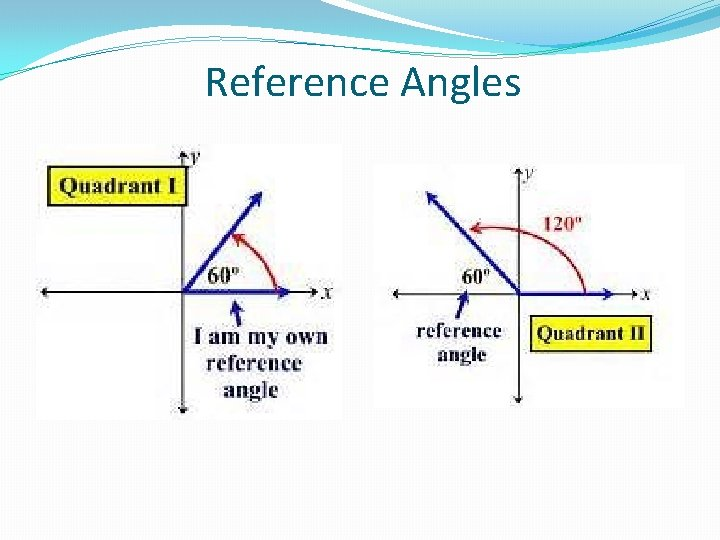Reference AnglesReference Angles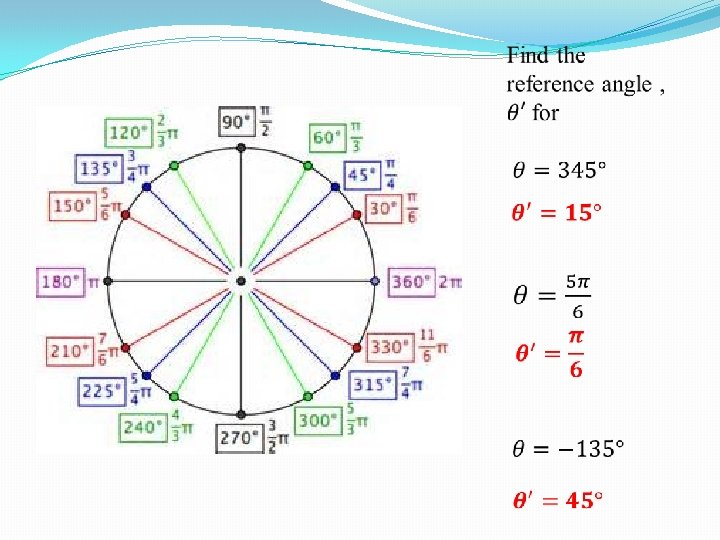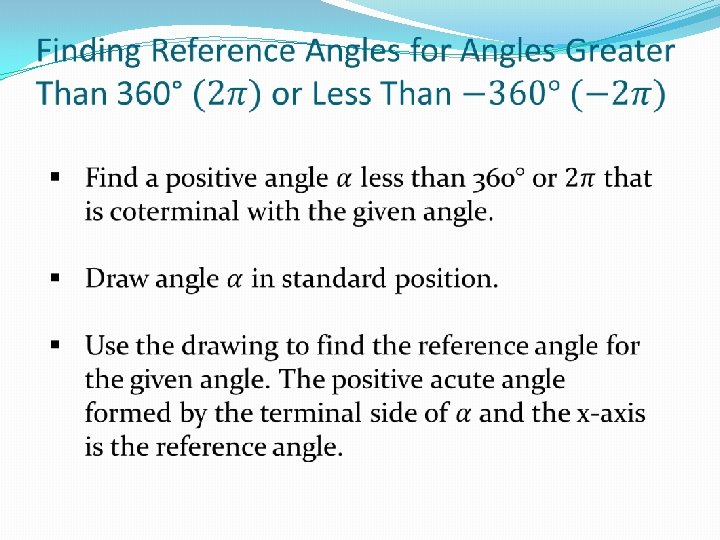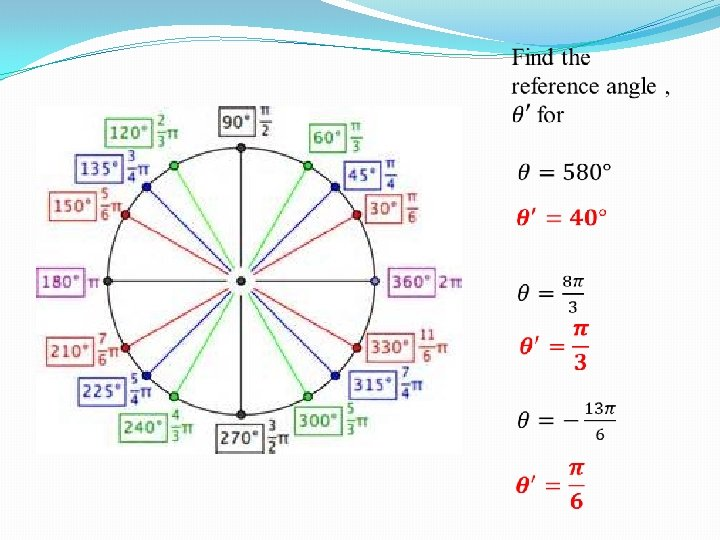Evaluating Trig Functions Using Reference Angles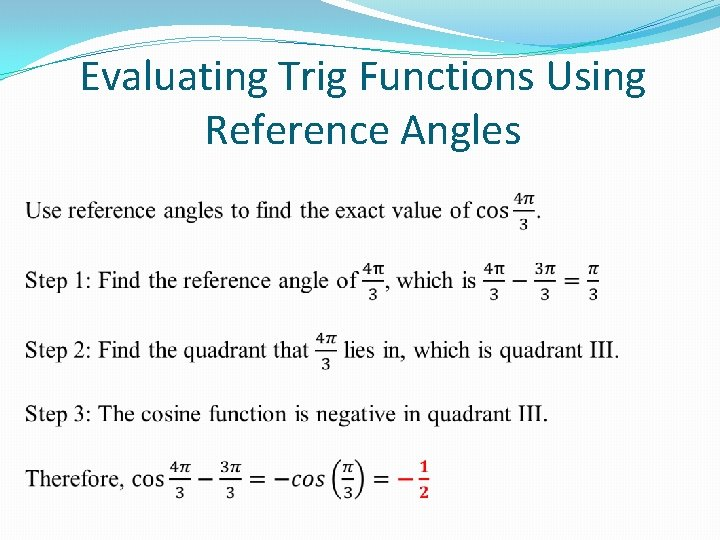Evaluating Trig Functions Using Reference AnglesEvaluating Trig Functions Using Reference Angles